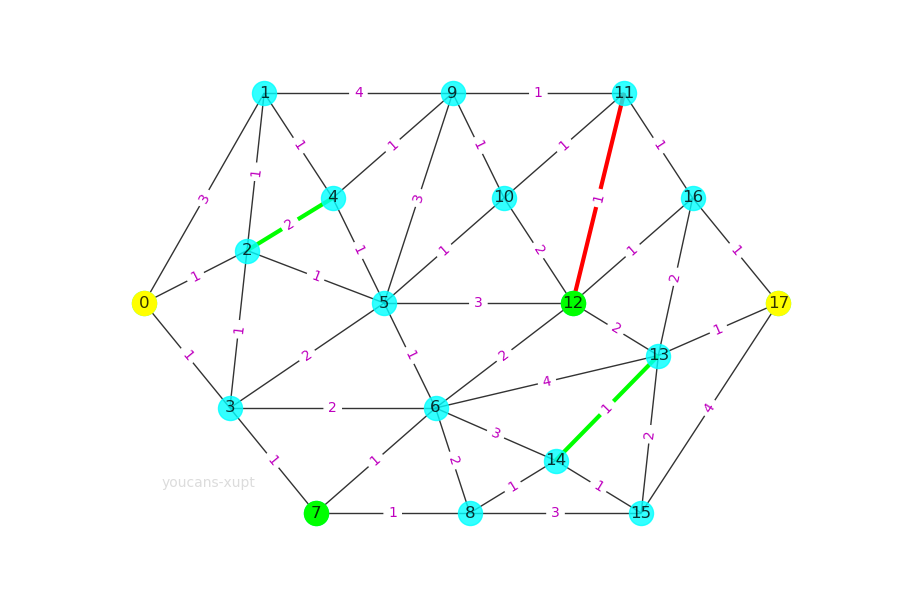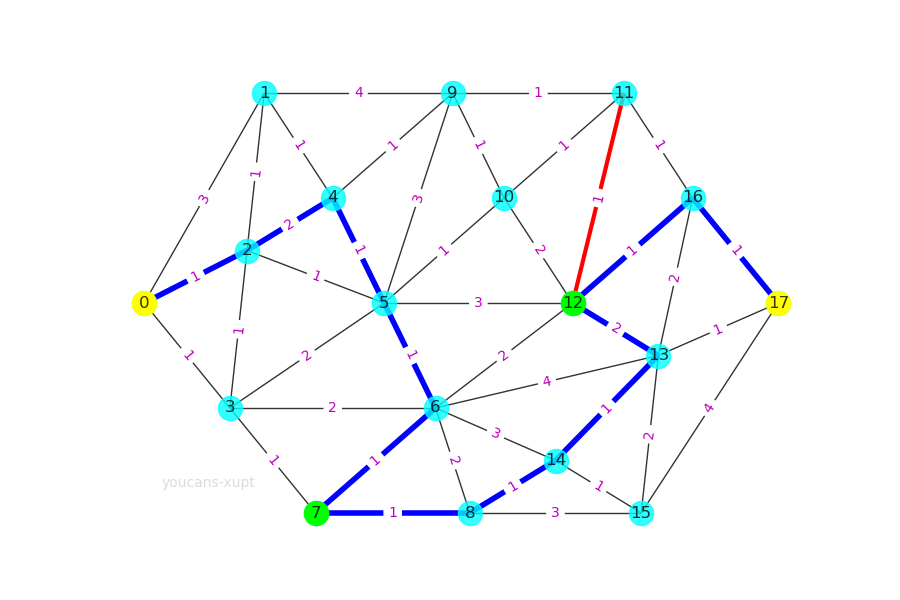# Python小白的数学建模课-17.条件最短路径

• 条件最短路径问题，指带有约束条件、限制条件的最短路径问题。例如： 顶点约束，包括必经点或禁止点的限制； 边的约束，包括必经路段、禁行路段和单向路段；无权路径长度的限制，如要求经过几步或不超过几步到达终点。
• 本文基于 NetworkX 工具包，建立了一个遍历简单路径、判断约束条件的通用框架。
• 数模竞赛真题案例，详解禁止点、禁止边、必经点、必经边的约束条件处理，进而可以扩展到任何约束条件。
• 『Python小白的数学建模课 @ Youcans』带你从数模小白成为国赛达人。

## 2. 案例：蚂蚁的最优路径分析

### 2.1 问题描述

• 必须经过图中的绿色节点 N7、N12；
• 必须经过图中的两段绿色路段 (N2, N4)、(N13, N14)；
• 必须避开图中的红色路段 (N11, N12)；
• 求花费最少的最优路径。### 2.2 图的创建和可视化

#### 2.2.1 Python 例程（NetworkX）

# mathmodel17_v1.py
# Demo17 of mathematical modeling algorithm
# Demo of shortest path with constraints with NetworkX
# Crated：2021-07-09

import numpy as np
import matplotlib.pyplot as plt # 导入 Matplotlib 工具包
import networkx as nx  # 导入 NetworkX 工具包

# 问题：蚂蚁的最优路径分析（西安邮电大学第12届数学建模竞赛B题）
gAnt = nx.Graph()  # 创建：空的 无向图
(1,2,1),(1,4,1),(1,9,4),
(2,3,1),(2,4,2),(2,5,1),
(3,5,2),(3,6,2),(3,7,1),
(4,5,1),(4,9,1),
(5,6,1),(5,9,3),(5,10,1),(5,12,3),
(6,7,1),(6,8,2),(6,12,2),(6,13,4),(6,14,3),
(7,8,1),
(8,14,1),(8,15,3),
(9,10,1),(9,11,1),
(10,11,1),(10,12,2),
(11,12,1),(11,16,1),
(12,13,2),(12,16,1),
(13,14,1),(13,15,2),(13,16,2),(13,17,1),
(14,15,1),
(15,17,4),
(16,17,1)])  # 向图中添加多条赋权边: (node1,node2,weight)

pos={0:(0,8),1:(7,12),2:(6,9),3:(5,6),4:(11,10),5:(14,8),  # 指定顶点位置
6:(17,6),7:(10,4),8:(19,4),9:(18,12),10:(21,10),11:(28,12),
12:(25,8),13:(30,7),14:(24,5),15:(29,4),16:(32,10),17:(37,8)}

fig, ax = plt.subplots(figsize=(9, 6))
nx.draw(gAnt, pos, with_labels=True, node_color='cyan', alpha=0.8)
labels = nx.get_edge_attributes(gAnt,'weight')  # 边的权值
nx.draw_networkx_edge_labels(gAnt,pos,edge_labels=labels, font_color='m')  # 显示边的权值
nx.draw_networkx_nodes(gAnt,pos,nodelist=[0,17],node_color='yellow')  # 设置顶点颜色：N1,N17
nx.draw_networkx_nodes(gAnt,pos,nodelist=[7,12],node_color='lime')  # 设置顶点颜色：N7,N12
nx.draw_networkx_edges(gAnt,pos,edgelist=[(2,4),(13,14)],edge_color='lime',width=3)  # 设置指定边的颜色、宽度
nx.draw_networkx_edges(gAnt,pos,edgelist=[(11,12)],edge_color='r',width=3)  # 设置指定边的颜色、宽度
plt.show()


#### 2.2.2 程序说明

1. 图的创建。本例使用 nx.Graph() 创建无向图，然后用 gAnt.add_weighted_edges_from() 函数以列表向图中添加多条赋权边，每个赋权边以元组 (node1,node2,weight) 表示。
2. 图的绘制。使用 nx.draw() 绘图时，默认的节点位置并不理想，可以使用 pos 属性参数指定节点位置。pos 为字典数据类型，按 node:(x_pos,y_pos) 格式设置节点位置。
3. 显示边的权值。使用 nx.draw_networkx_edge_labels() 可以绘制边的属性，例程显示权值属性 'weight'。
4. 设置顶点属性。nx.draw_networkx_nodes() 可以设置顶点的属性，例如对 nodelist 列表中的节点设置颜色属性 node_color。
5. 设置边的属性。nx.draw_networkx_edges() 可以设置边的属性，例如对 edgelist 列表中的边设置线宽属性 width 和颜色属性 edge_color。

## 3. NetworkX 求解条件最短路径问题

### 3.1 无限制条件的最短路径

Python 例程（NetworkX）：

# 1. 无限制条件的最短路径
# Dijkstra 算法：两个指定顶点之间的最短加权路径
minWPath1 = nx.dijkstra_path(gAnt, source=0, target=17)  # 顶点 0 到 顶点 17 的最短加权路径
# Dijkstra 算法：两个指定顶点之间的最短加权路径的长度
lMinWPath1 = nx.dijkstra_path_length(gAnt, source=0, target=17)  # 最短加权路径长度
print("\n问题1: 无限制条件")
print("N0 到 N17 的最短加权路径: ", minWPath1)
print("N0 到 N17 的最短加权路径长度: ", lMinWPath1)


问题1: 无限制条件
N0 到 N17 的最短加权路径:  [0, 2, 5, 10, 11, 16, 17]
N0 到 N17 的最短加权路径长度:  6


1. 对于无限制条件的最短路径问题，NetworkX 提供了 Dijkstra 算法、Bellman-Ford 算法、Floyd 算法和启发式算法 A* 的函数。
2. 例程使用 nx.dijkstra_path() 和 nx.dijkstra_path_length() 调用 Dijkstra 算法求两个指定顶点之间的最短加权路径和最短加权路径长度。

### 3.2 限制条件：禁止点或禁止边

1. 禁止点或者禁止边的处理比较简单，从图中删除对应的禁止顶点或禁止边即可。当然，也可以在创建图时就不添加这些禁止点和禁止边，但这会导致绘图时也无法反映这些顶点和边。
2. 使用 remove_node(n) 删除指定顶点 n，remove_edge(u,v) 删除指定的边 (u,v)。
3. 使用 remove_nodes_from([n1,...nk]) 删除多个顶点，remove_edges_from([(u1,v1),...(uk,vk)]) 删除多条边。
4. 本例程中删除的点和边与 2.1 的问题描述中的要求不一致，是为了示例删除函数的使用。下同。

Python 例程：

# 2. 限制条件：禁止点或禁止边
# 解决方案：从图中删除禁止顶点或禁止边
gAntF = gAnt.copy()
gAntF.remove_node(5)  # 通过顶点标签 5 删除顶点
gAntF.remove_edges_from([(11,12), (13,17)])  # 删除多条边 (11,12), (13,17)
minWPath2 = nx.dijkstra_path(gAntF, source=0, target=17)  # 顶点 0 到 顶点 17 的最短加权路径
lMinWPath2 = nx.dijkstra_path_length(gAntF, source=0, target=17)  # 最短加权路径长度
print("\n问题2: 禁止点或禁止边的约束")  # youcans @ XUPT
print("N0 到 N17 的最短加权路径: ", minWPath2)
print("N0 到 N17 的最短加权路径长度: ", lMinWPath2)


问题2: 禁止点或禁止边的约束
N0 到 N17 的最短加权路径:  [0, 3, 6, 12, 16, 17]
N0 到 N17 的最短加权路径长度:  7


### 3.3 限制条件：一个必经点

1. 当限制条件为一个必经点时，可以把原问题分解为两个子问题：子问题 1 为起点至必经点，子问题 2 为必经点至终点。
2. 对两个子问题分别求其最短加权路径和最短加权路径长度，然后将两个子问题的结果合并，就得到经过必经点的原问题的最短加权路径和最短加权路径长度。

Python 例程：

# 3. 限制条件：一个必经点
# 解决方案：分解为两个问题，问题 1 为起点N0至必经点N6，问题 2 为必经点N6至终点N17
gAntP = gAnt.copy()
minWPath3a = nx.dijkstra_path(gAntP, source=0, target=6)  # N0 到 N6 的最短加权路径
lMinWPath3a = nx.dijkstra_path_length(gAntP, source=0, target=6)  # 最短加权路径长度
minWPath3b = nx.dijkstra_path(gAntP, source=6, target=17)  # N6 到 N17 的最短加权路径
lMinWPath3b = nx.dijkstra_path_length(gAntP, source=6, target=17)  # 最短加权路径长度
minWPath3a.extend(minWPath3b[1:]) # 拼接 minWPath3a、minWPath3b 并去重 N7
print("\n问题3: 一个必经点(N6)的约束")
print("N0 经 N6 到 N17 的最短加权路径: ", minWPath3a)
print("N0 经 N6 到 N17 的最短加权路径长度: ", lMinWPath3a+lMinWPath3b)


问题3: 一个必经点(N6)的约束
N0 经 N6 到 N17 的最短加权路径:  [0, 3, 6, 12, 16, 17]
N0 经 N6 到 N17 的最短加权路径长度:  7


### 3.4 限制条件：多个必经点（方案一）

1. 当限制条件为两个或多个必经点，如果指定这些必经点的先后顺序，可以按 3.3 的方法将原问题分解为多个子问题。
2. 如果不指定这些比较点的先后顺序， 从起点出发不知道应该先去哪个必经点，这是有两种处理方法：一是用穷举法对所有可能的顺序都进行计算，然后进行比较；二是通过约束条件对所有简单路径进行判断。
3. NetworkX 提供了 all_simple_paths() 函数，可以生成两个顶点之间的所有简单路径。利用简单路径算法，可以通过对约束条件的判断来求解带有多个顶点约束的最短路径问题。
4. 程序实现的步骤包括：
• （1）生成指定起点、终点的所有简单路径；
• （2）判断路径是否满足包括所有必经点的限制条件；
• （3）在满足限制条件的简单路径中找加权长度最短的路径；
• （4）求最短路径的加权路径长度。
1. 本段例程非常简练，综合使用了几种 Python 语言循环、判断结构的简洁写法，已经逐句注释分析，读者需要认真研读。

Python 例程：

# 4. 限制条件：多个必经点 (N7,N15)
# 解决方案：遍历从起点到终点的简单路径，求满足必经点条件的最短路径
gAntM = gAnt.copy()
minWPath4 = min([path  # 返回 key 为最小值的 path
for path in nx.all_simple_paths(gAntM, 0, 17)  # gAnt 中所有起点为0、终点为17的简单路径
if all(n in path for n in (7, 15))],  # 满足路径中包括顶点 N7,N15
key=lambda x: sum(gAntM.edges[edge]['weight'] for edge in nx.utils.pairwise(x)))  # key 为加权路径长度
lenPath4 = sum(gAntM.edges[edge]['weight'] for edge in nx.utils.pairwise(minWPath4))  # 求指定路径的加权路径长度
print("\n问题4: 多个必经点(N7, N15)的约束")
print("N0 经 N7, N15 到 N17 的最短加权路径: ", minWPath4)
print("N0 经 N7, N15 到 N17 的最短加权路径长度: ", lenPath4)


问题4: 多个必经点(N7, N15)的约束
N0 经 N7, N15 到 N17 的最短加权路径:  [0, 3, 7, 8, 14, 15, 13, 17]
N0 经 N7, N15 到 N17 的最短加权路径长度:  8



### 3.5 限制条件：多个必经点（方案二）

1. 本例与 3.5 的问题实际上是相同的。限制条件都是多个必经顶点 N7、N15，解决方案都是使用 all_simple_paths() 函数生成两个顶点间的所有简单路径，程序实现的步骤也是类似的。
2. 本方案按照典型的循环、判断结构的写法，便于阅读和理解。此外，如果还有其它约束条件或子任务需要在循环中处理，这样的结构更容易实现。

Python 例程

# 5. 限制条件：多个必经点 (N7,N15)
# 解决方案：多重循环、判断结构，遍历从起点到终点的简单路径，求满足必经点条件的最短路径
gAntM = gAnt.copy()
lMinWPath5 = minWPath5 = 1e9
for path in nx.all_simple_paths(gAntM, 0, 17):
if all(n in path for n in (7,15)):  # 满足路径中包括顶点 N7,N15
lenPath = sum(gAntM.edges[edge]['weight'] for edge in nx.utils.pairwise(path))
if lenPath < lMinWPath5:
lMinWPath5 = lenPath
minWPath5 = path
print("\n问题5: 多个必经点(N7, N15)的约束")
print("N0 经 N7, N15 到 N17 的最短加权路径: ", minWPath5)
print("N0 经 N7, N15 到 N17 的最短加权路径长度: ", lMinWPath5)



问题5: 多个必经点(N7, N15)的约束
N0 经 N7, N15 到 N17 的最短加权路径:  [0, 3, 7, 8, 14, 15, 13, 17]
N0 经 N7, N15 到 N17 的最短加权路径长度:  8



### 3.6 限制条件：必经边

1. 延续处理多个必经点的思路，生成两个顶点之间的所有简单路径，通过对必经边约束条件的判断来求解必经边约束最短路径问题。
2. 本例程的框架和步骤同 3.5，按照典型的循环、判断结构的写法，便于阅读和理解。
3. all(n in path for n in (2,4,13,14)) 的作用，是判断路径中是否包括必经边 (2,4)、(13,14) 的各顶点，这不仅可以减小计算量，而且能确保下面使用 index() 查找顶点位置时不会发生错误。

Python 例程

# 6. 限制条件：必经边 (N2,N4), (N13,N14)
# 解决方案：遍历从起点到终点的简单路径，求满足必经边条件的最短路径
gAntE = gAnt.copy()
lMinWPath6 = minWPath6 = 1e9  # 置初值
for path in nx.all_simple_paths(gAntE, 0, 17):  # 所有起点为0、终点为17的简单路径
if all(n in path for n in (2,4,13,14)):  # 满足路径中包括必经边的顶点 N2,N4,N13,N14
# 检查 (N2,N4)
p1 = path.index(2)  # N2 的位置
if (path[p1-1]!=4 and path[p1+1]!=4): continue  # 判断 N2~N4 是否相邻
# 检查 (N13,N14)
p2 = path.index(13)  # # N13 的位置
if (path[p2-1]!=14 and path[p2+1]!=14): continue  # 判断 N13~N14 是否相邻
lenPath = sum(gAntE.edges[edge]['weight'] for edge in nx.utils.pairwise(path))
if lenPath < lMinWPath6:
lMinWPath6 = lenPath
minWPath6 = path
print("\n问题6: 必经边 (N2,N4), (N13,N14) 的约束")
print("N0 到 N17 的最短加权路径: ", minWPath6)
print("N0 到 N17 的最短加权路径长度: ", lMinWPath6)



问题6: 必经边 (N2,N4), (N13,N14) 的约束
N0 到 N17 的最短加权路径:  [0, 2, 4, 5, 6, 7, 8, 14, 13, 17]
N0 到 N17 的最短加权路径长度:  10



## 4. NetworkX 求解蚂蚁最优路径问题

### 4.1 程序说明

1. 对于必经点的处理，实际上还可以有更好的方法，即结合 Dijkstra 算法的实现过程， 将限制条件作为缩小搜索空间的条件，可以降低算法的复杂度。但对于多个必经边来说，很难以此来改进基础的无约束算法，通常的处理方法就是在算法中增加一个判断是否满足约束条件的过程。
2. 本例程生成两个顶点之间的所有简单路径，通过对各种约束条件的判断来求解必经边约束最短路径问题，可以同时处理禁止点、禁止边、必经点、必经边的约束条件。
3. 本例程对应案例中的各项约束条件： 必须经过图中的绿色节点；必须经过图中的两段绿色路段；必须避开图中的红色路段；尽可能以最少的花费到达终点。
4. 本例程是一个遍历简单路径、判断约束条件的通用框架。
5. all(n in path for n in (2,4,7,12,13,14)) 的作用，一是判断路径中是否包括必经点 N7、N12；二是判断路径中是否包括必经边 (2,4)、(13,14) 的各顶点，这不仅可以减小计算量，而且能确保下面使用 index() 查找顶点位置时不会发生错误。

### 4.2 Python 例程

# mathmodel17_v1.py
# Demo17 of mathematical modeling algorithm
# Demo of shortest path with constraints with NetworkX
# Crated：2021-07-09

import numpy as np
import matplotlib.pyplot as plt # 导入 Matplotlib 工具包
import networkx as nx  # 导入 NetworkX 工具包

# 问题：蚂蚁的最优路径分析（西安邮电大学第12届数学建模竞赛B题）
gAnt = nx.Graph()  # 创建：空的 无向图
(1,2,1),(1,4,1),(1,9,4),
(2,3,1),(2,4,2),(2,5,1),
(3,5,2),(3,6,2),(3,7,1),
(4,5,1),(4,9,1),
(5,6,1),(5,9,3),(5,10,1),(5,12,3),
(6,7,1),(6,8,2),(6,12,2),(6,13,4),(6,14,3),
(7,8,1),
(8,14,1),(8,15,3),
(9,10,1),(9,11,1),
(10,11,1),(10,12,2),
(11,12,1),(11,16,1),
(12,13,2),(12,16,1),
(13,14,1),(13,15,2),(13,16,2),(13,17,1),
(14,15,1),
(15,17,4),
(16,17,1)])  # 向图中添加多条赋权边: (node1,node2,weight)

pos={0:(0,8),1:(7,12),2:(6,9),3:(5,6),4:(11,10),5:(14,8),  # 指定顶点位置
6:(17,6),7:(10,4),8:(19,4),9:(18,12),10:(21,10),11:(28,12),
12:(25,8),13:(30,7),14:(24,5),15:(29,4),16:(32,10),17:(37,8)}

fig, ax = plt.subplots(figsize=(9, 6))
nx.draw(gAnt, pos, with_labels=True, node_color='cyan', alpha=0.8)
labels = nx.get_edge_attributes(gAnt,'weight')  # 边的权值
nx.draw_networkx_edge_labels(gAnt,pos,edge_labels=labels, font_color='m')  # 显示边的权值
nx.draw_networkx_nodes(gAnt,pos,nodelist=[0,17],node_color='yellow')  # 设置顶点颜色：N1,N17
nx.draw_networkx_nodes(gAnt,pos,nodelist=[7,12],node_color='lime')  # 设置顶点颜色：N7,N12
nx.draw_networkx_edges(gAnt,pos,edgelist=[(2,4),(13,14)],edge_color='lime',width=3)  # 设置指定边的颜色、宽度
nx.draw_networkx_edges(gAnt,pos,edgelist=[(11,12)],edge_color='r',width=3)  # 设置指定边的颜色、宽度

# 7. 限制条件：必经点 N7,N12，必经边 (N2,N4), (N13,N14)，禁止边 (11,12)
# 解决方案：遍历从起点到终点的简单路径，求满足必经边条件的最短路径
gAntS = gAnt.copy()
gAntS.remove_edge(11,12)  # 删除禁止边 (11,12)
lMinWPath = minWPath = 1e9  # 置初值
for path in nx.all_simple_paths(gAntS, 0, 17):  # 所有起点为0、终点为17的简单路径
if all(n in path for n in (2,4,7,12,13,14)): # 满足路径中包括顶点 N7,N12
# 检查 (N2,N4)
p1 = path.index(2)  # N2 的位置
if (path[p1-1]!=4 and path[p1+1]!=4): continue  # 判断 N2~N4 是否相邻
# 检查 (N13,N14)
p2 = path.index(13)  # # N13 的位置
if (path[p2-1]!=14 and path[p2+1]!=14): continue  # 判断 N13~N14 是否相邻
lenPath = sum(gAntS.edges[edge]['weight'] for edge in nx.utils.pairwise(path))
if lenPath < lMinWPath:
lMinWPath = lenPath
minWPath = path

print("\n蚂蚁最优路径问题（带有禁止点、禁止边、必经点、必经边的约束条件）")
print("约束条件：必经点 N7,N12，必经边 (N2,N4), (N13,N14)，禁止边 (11,12)")
print("N0 到 N17 的最短加权路径: ", minWPath)
print("N0 到 N17 的最短加权路径长度: ", lMinWPath)

edgeList = []
for i in range(len(minWPath)-1):
edgeList.append((minWPath[i],minWPath[i+1]))
nx.draw_networkx_edges(gAnt,pos,edgelist=edgeList,edge_color='b',width=4)  # 设置边的颜色
plt.show()



### 4.3 运行结果

蚂蚁最优路径问题（带有禁止点、禁止边、必经点、必经边的约束条件）

N0 到 N17 的最短加权路径:  [0, 2, 4, 5, 6, 7, 8, 14, 13, 12, 16, 17]
N0 到 N17 的最短加权路径长度:  13## 5. 总结

1. 数模竞赛中的最短路径问题，往往是带有约束条件的最短路径问题。
2. 很多计算机语言算法工具包（包括但不限于 Python）都对算法进行了封装，没有提供设置约束条件的选项和接口，因此用户不能把条件判断语句加入这些封装函数的程序内部。
3. 本文基于 NetworkX 工具包，建立了一个遍历简单路径、判断约束条件的通用框架，不仅可以处理禁止点、禁止边、必经点、必经边的约束条件处理，而且可以扩展到任何约束条件。

【本节完】